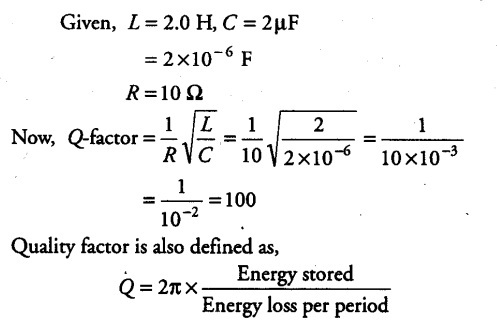# Calculate the quality factor of a series

Calculate the quality factor of a series L- C- R circuit with L= 2.0 H ,C = 2 pF and R = 10 £2. Mention the significance of quality factor in L-C-R circuit.So, higher the value of Q means the energy loss is at lower rate relative to the energy stored, i.e. the oscillations will die slowly and damping would be less.